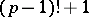# Difference between revisions of "Wilson theorem"

Letbe a prime number. Then the numberis divisible by. The theorem was first formulated by E. Waring (1770) and is, according to him, due to J. Wilson. It was proved by J.L. Lagrange in 1771. A primality test for integers follows from Wilson's theorem: A natural numberwill be prime if and only if